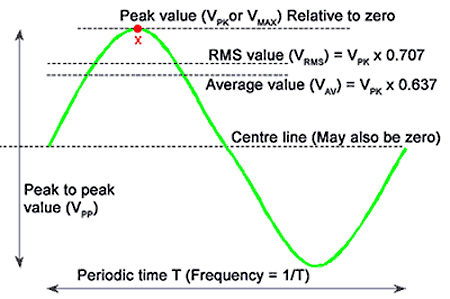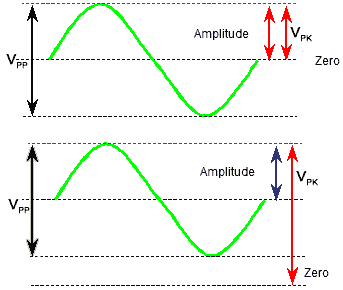# Measuring the Sine Wave.

• • Know measurements associated with sine waves
• • a. Peak to peak value.
• • b. Amplitude.
• • c. Peak value.
• • d. Periodic time.
• • e. Average value.
• • f. RMS value.### Fig 1.2.1 Characteristics of a Sine Wave

A wave form is a graph showing the variation, usually of voltage or current, against time. The horizontal axis shows the passing of time, progressing from left to right. The vertical axis shows the quantity measured (this is voltage in Fig 1.2.1).

Six of the most important characteristics of a sine wave are;

PEAK TO PEAK value.

INSTANTANEOUS value.

AMPLITUDE.

PEAK value.

PERIODIC TIME.

AVERAGE value.

RMS value.

These characteristics are illustrated in fig 1.2.1

## Peak to Peak Value

The PEAK TO PEAK value is the vertical distance between the top and bottom of the wave. It will be measured in volts on a voltage waveform, and may be labelled VPP or VPK−PK. In a current waveform it would be labelled IPP or IPK−PK as I (not C) is used to represent current.

## Instantaneous Value

This is the value (voltage or current) of a wave at any particular instant. often chosen to coincide with some other event. E.g. The instantaneous value of a sine wave one quarter of the way through the cycle will be equal to the peak value. See point X in Fig 1.2.1.

## Amplitude

The AMPLITUDE of a sine wave is the maximum vertical distance reached, in either direction from the centre line of the wave. As a sine wave is symmetrical about its centre line, the amplitude of the wave is half the peak to peak value, as shown in Fig 1.2.2.

## Peak Value

The PEAK value of the wave is the highest value the wave reaches above a reference value. The reference value normally used is zero. In a voltage waveform the peak value may be labelled VPK or VMAX (IPK or IMAX in a current waveform).

If the sine wave being measured is symmetrical either side of zero volts (or zero amperes), meaning that the dc level or dc component of the wave is zero volts, then the peak value must be the same as the amplitude, that is half of the peak to peak value.### Fig 1.2.2 Defining the Peak value VPK

However this is not always the case, if a dc component other than zero volts is also present, the sine wave will be symmetrical about this level rather than zero. The bottom waveform in Fig 1.2.2 shows that the peak value can now be even larger than the peak to peak value, (the amplitude of the wave however, remains the same, and is the difference between the peak value and the "centre line" of the waveform).

## Periodic Time & Frequency

The PERIODIC TIME (given the symbol T) is the time, in seconds milliseconds etc. taken for one complete cycle of the wave. It can be used to find the FREQUENCY of the wave ƒ using the formula T =1/ƒ

Thus if the periodic time of a wave is 20ms (or 1/50th of a second) then there must be 50 complete cycles of the wave in one second. A frequency of 50 Hz. Note that when you use this formula, if the periodic time is in seconds then the frequency will be in Hz.## Average Value

The AVERAGE value. This is normally taken to mean the average value of only half a cycle of the wave. If the average of the full cycle was taken it would of course be zero, as in a sine wave symmetrical about zero, there are equal excursions above and below the zero line.

Using only half a cycle, as illustrated in fig 1.2.3 the average value (voltage or current) is always 0.637 of the peak value of the wave.

VAV = VPK x 0.637

or

IAV = IPK X 0.637

The average value is the value that usually determines the voltage or current indicated on a test meter. There are however some meters that will read the RMS value, these are called "True RMS meters".

## The RMS Value.

The RMS or ROOT MEAN SQUARED value is the value of the equivalent direct (non varying) voltage or current which would provide the same energy to a circuit as the sine wave measured. That is, if an AC sine wave has a RMS value of 240 volts, it will provide the same energy to a circuit as a DC supply of 240 volts.

It can be shown that the RMS value of a sine wave is 0.707 of the peak value.

VRMS = VPK x 0.707   and   IRMS = IPK x 0.707

Also, the peak value of a sine wave is equal to 1.414 x the RMS value.

## The Form Factor

If VAV (0.637) is multiplied by 1.11 the answer is 0.707, which is the RMS value. This difference is called the Form Factor of the wave, and the relationship of 1.11 is only true for a perfect sine wave. If the wave is some other shape, either the RMS or the average value (or both) will change, and so will the relationship between them. This is important when measuring AC voltages with a meter as it is the average value that most meters actually measure. However they display the RMS value simply by multiplying the voltage by 1.11. Therefore if the AC wave being measured is not a perfect sine wave the reading will be slightly wrong. If you pay enough money however, you can buy a true RMS meter that actually calculates the RMS value of non-sine waves.

## The Mains (Line) Supply

To demonstrate some of these characteristics in use, consider a very common sine wave, the mains supply or line waveform, which in many parts of the world is a nominal 230V.Electrical equipment that connects to the mains supply always carries a label giving information about what supply the equipment can be connected to. These labels are quite variable in appearance, but often there is a picture of a sine wave showing that an a.c. supply must be used. The voltage quoted will be 230V (or 120V in the USA)or range of voltages including these values. These voltages actually refer to the RMS value of the mains sine wave. The label also states that the frequency of the supply, which is 50Hz in Europe or 60Hz in the USA.

From this small amount of information other values can be worked out:

a. The peak voltage of the waveform, as VPK = VRMS x 1.414

b. The AVERAGE value of the waveform, as VAV = VPK x 0.637

c. The PEAK TO PEAK value of the waveform. This is twice the AMPLITUDE, which (because the mains waveform is symmetrical about zero volts) is the same value as VPK.

Because VPK is already known from a. it follows that VPP = VPK x 2

d.The PERIODIC TIME which is given by T =1/ƒ

Top of Page.>